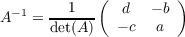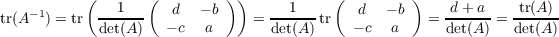## Math 501: Differential Geometry

Homework 7 <me@tylerlogic.com>

April 7, 2013
http://coursework.tylerlogic.com/courses/math501/homework07

1

Taken off the homework.

2

3 do Carmo pg 212 problem 11

Let X be a parametrization of a surface with normal N. Define Y to be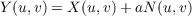(3.1)

for some positive a.

(a)

We know the following to hold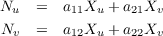where (aij) is the matrix representation of the differential of N. section 3.1 yields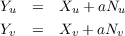and thus combining the two sets of equations above we are left with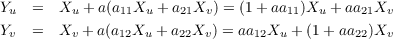With this, we can take the cross product of Y u and Y v revealing that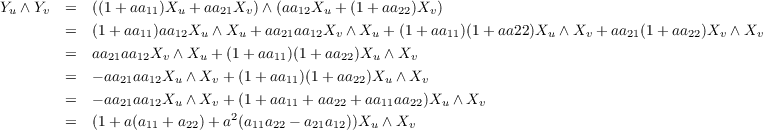Now since K = det([dN]) and H = -12tr([dN]) for Gaussian and mean curvatures of X, then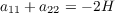and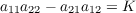resulting in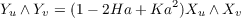(b)

Let F be the homeomorphism from S to the parallel surface defined by F(p) = p + aN(p). Thus we have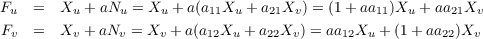indicating that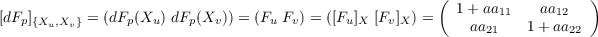which results in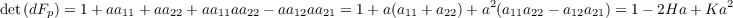(3.2)

Now because of part (a), we know that the normal field for the parallel surface, call it M, at F(p) is the same as the normal field for S at p, i.e.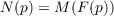(3.3)

We will make use of this to determine the Gaussian and mean curvatures.

Gaussian Curvature Using the chain rule with subsection 3.3 gives us that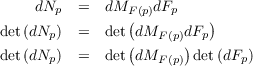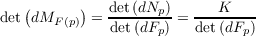(3.4)

The combination of subsection 3.2 and paragraph 3.4 leads to the parallel surface having a Gaussian curvature, K, of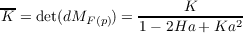Mean Curvature Again using the chain rule with subsection 3.3 and employing Lemma A.1 and paragraph 3.4 we find that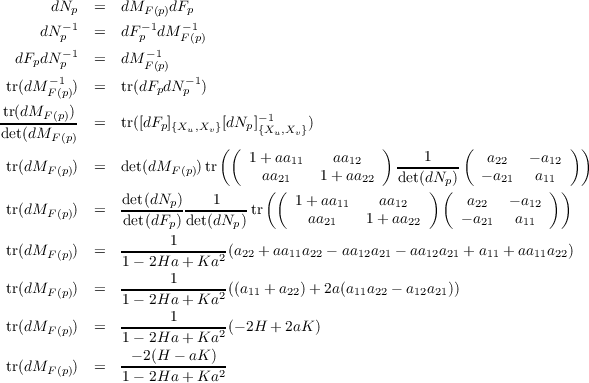Hence the mean curvature, H, is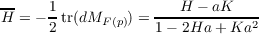(c)

4 do Carmo pg 229 problem 9

Let S1 and S2 be regular surfaces with a conformal maps φ : S1 S2 and ψ : S2 S3.

(a) Inverses of isometries are isometries

The proof in Problem section 8 part (a) holds for this when λ is the constant function of 1.

(b) Composition of isometries is an isometry

The proof in Problem section 8 part (b) holds for this when λφ and λψ are both the constant functions of 1.

5 do Carmo pg 229 problem 10

Let φ : S S be a rotation about the axis of a surface of revolution, S. Because it is simply a rotation, φ is the restriction of some linear map of rotation, R : 3 3, to S. Hence for v S, φ(p) = Ap for some matrix of rotation A. Note that rotational matrices such as A are orthogonal. Thus we have the following for p S and v TpS with some curve α such that α(0) = p and α(0) = v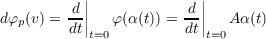but because A is not dependent on t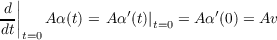resulting in p(v) = Av. Thus since A is orthogonal then for any v,w TpS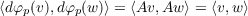thereby giving us that φ is an isometry.

6

(a)

The drawing in Figure 1 has two points p and q in a planar surface for which there is no curve between them with length equal to the intrinsic distance between the two points. A curve that would potentially have a length of the intrinsic distance would need to go through the hole in the middle of the surface, but it obviously cannot while remaining a curve of the surface.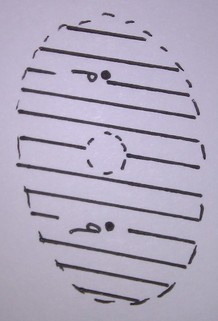Figure 1: A planar surface with two points for no curve between the two has a length equal to the intrinsic distance.

(b)

From our first homework assignment, we know that for any curve α in S with α(a) = p and α(b) = q, L(α)ab ≥|p-q|. Thus because d(p,q) is the infimum a set of the lengths (from a to b) of curves in S which pass through p and q at a and b, respectively, then d(p,q) ≥|p - q|.

(c)

7

(a) Coefficients of the first fundamental form.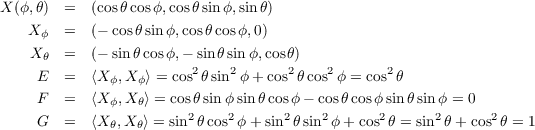(b) Relation of M,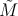, X, and Y

Since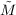is the map from the domain of X to the domain of Y , then we have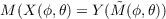(7.5)

for (ϕ,θ) in the domain of X.

(c) Coefficients of the first fundamental form of X

By subsection 7.5 we have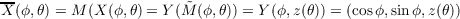which results in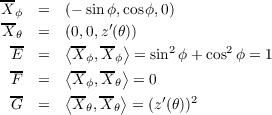(d)

In order to have M be a conformal map, we must satisfy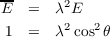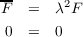and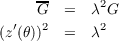indicating that z(θ) must be secθ.

(e)

From the result of the previous part of the problem we know that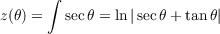assuming z(0) = 0 and z(θ) > 0 for θ (0,π∕2).

8

Let S1 and S2 be regular surfaces with a conformal maps φ : S1 S2 and ψ : S2 S3.

(a) Inverses of conformal maps are conformal

Since S1 and S2 are diffeomorphic, then TpS1 = Tφ(p)S2 and p = φ(p)-1 for p S2. Thus for vectors v1,v2 TpS2 = Tφ-1(p)S1, the vectors p-1(v1) and p-1(v2) are vectors in Tp(S1). So we have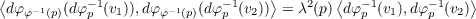which in turn implies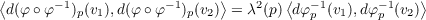however, φ φ-1 is the identity map implying that the above equation simplifies to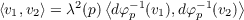which gives us what we’re looking for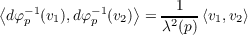(b) Composition of conformal maps is conformal

Let p S1 and v1,v2 TpS. Then we have the following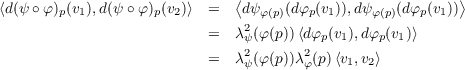so for λ(p) = λψ(φ(p))λφ(p) we have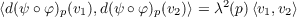giving us the fact that φ ψ is conformal since it is a diffeomorphism.

(c)

# Appendix

Lemma A.1 The trace of the inverse of a two-dimensional matrix A is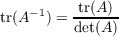Proof. Let A be the matrix denoted by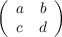then